MAT331 homework problems
13.
(expires 11 March)     Find all the solutions to the differential equation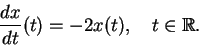Among them, single out the one for which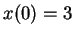. [Hint: read the help page for dsolve, or just do it in your head. It is that easy.]

14.
(expires 11 March)     Have Maple find analytic solutions to the following system of differential equations,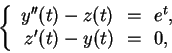with initial conditions: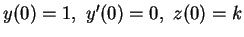. Let us denote the solutions by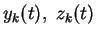(since they depend on the parameter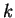).

Fortaking all integer values from -10 to 10, and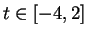, plot the functions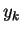in blue, and the functions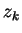in red, all on the same graph. (Yes, you will then have 42 functions plotted on the same graph.) [This is certainly a case when you don't want to retype the functions that Maple finds. You will almost certainly need to read the help page for dsolve. I also found subs, unapply, and seq useful.]

15.
(expires 11 March)     For the functions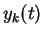and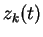found in problem #14, plot the parametric curves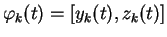for integer values ofbetween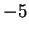and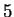and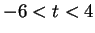on the same graph. Use the view option of plot to only show what lies in the region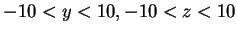, and use a sequence of colors so that each solution is a different color. [ HINT: you might find something like seq(COLOR(HUE,i/11),i=0..10) useful for the latter.]

16.
(expires 11 March)     Find all the fixed points of the system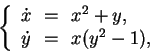a fixed point being a solution for which both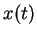and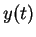stay constant. For each of these points, describe the behavior of the solutions that have initial conditions nearby. You can use Maple to figure out what happens for nearby points, or you can use more mathematical methods.

NOTE: The fact that there are various notations for differential equations is purely intentional.

MAT 331 2002-03-07